# 6. SPARK Tutorial¶

This chapter describes a simple use of the SPARK toolset on a program written completely in SPARK, within the GNAT Studio integrated development environment. All the tools may also be run from the command-line, see Command Line Invocation.

Note

If you’re using SPARK Discovery instead of SPARK Pro, some of the proofs in this tutorial may not be obtained automatically. See the section on Alternative Provers to install additional provers that are not present in SPARK Discovery.

## 6.1. Writing SPARK Programs¶

As a running example, we consider a naive searching algorithm for an unordered collection of elements. The algorithm returns whether the collection contains the desired value, and if so, at which index. The collection is implemented here as an array. We deliberately start with an incorrect program for package `Search`, in order to explain how the SPARK toolset can help correct these errors. The final version of the `linear_search` example is part of the Examples in the Toolset Distribution.

We start with creating a GNAT project file in `search.gpr`:

 ```1 2 3 4 5 6 7``` ```project Search is for Source_Dirs use ("."); package Compiler is for Switches ("Ada") use ("-gnatwa"); end Compiler; end Search; ```

It specifies that the source code to inspect is in the current directory, and that the code should be compiled at maximum warning level (switch `-gnatwa`). GNAT projects are used by most tools in the GNAT toolsuite; for in-depth documentation of this technology, consult the GNAT User’s Guide. Documentation and examples for the SPARK language and tools are also available via the Help ‣ SPARK menu in GNAT Studio.

The obvious specification of `Linear_Search` is given in file `linear_search.ads`, where we specify that the spec is in SPARK by using aspect `SPARK_Mode`.

 ``` 1 2 3 4 5 6 7 8 9 10 11 12 13 14 15 16``` ```package Linear_Search with SPARK_Mode is type Index is range 1 .. 10; type Element is new Integer; type Arr is array (Index) of Element; function Search (A : Arr; Val : Element; At_Index : out Index) return Boolean; -- Returns True if A contains value Val, in which case it also returns -- in At_Index the first index with value Val. Returns False otherwise. end Linear_Search; ```

The implementation of `Linear_Search` is given in file `linear_search.adb`, where we specify that the body is in SPARK by using aspect `SPARK_Mode`. It is as obvious as its specification, using a loop to go through the array parameter `A` and looking for the first index at which `Val` is found, if there is such an index.

 ``` 1 2 3 4 5 6 7 8 9 10 11 12 13 14 15 16 17 18 19 20 21 22 23 24``` ```package body Linear_Search with SPARK_Mode is function Search (A : Arr; Val : Element; At_Index : out Index) return Boolean is Pos : Index := A'First; begin while Pos < A'Last loop if A(Pos) = Val then At_Index := Pos; return True; end if; Pos := Pos + 1; end loop; return False; end Search; end Linear_Search; ```

We can check that the above code is valid Ada by using the ```Build > Check Semantic``` menu, which completes without any errors or warnings:### 6.1.1. Checking SPARK Legality Rules¶

Now, let us run GNATprove on this unit, using the SPARK ‣ Examine File menu, so that it issues errors on SPARK code that violates SPARK rules: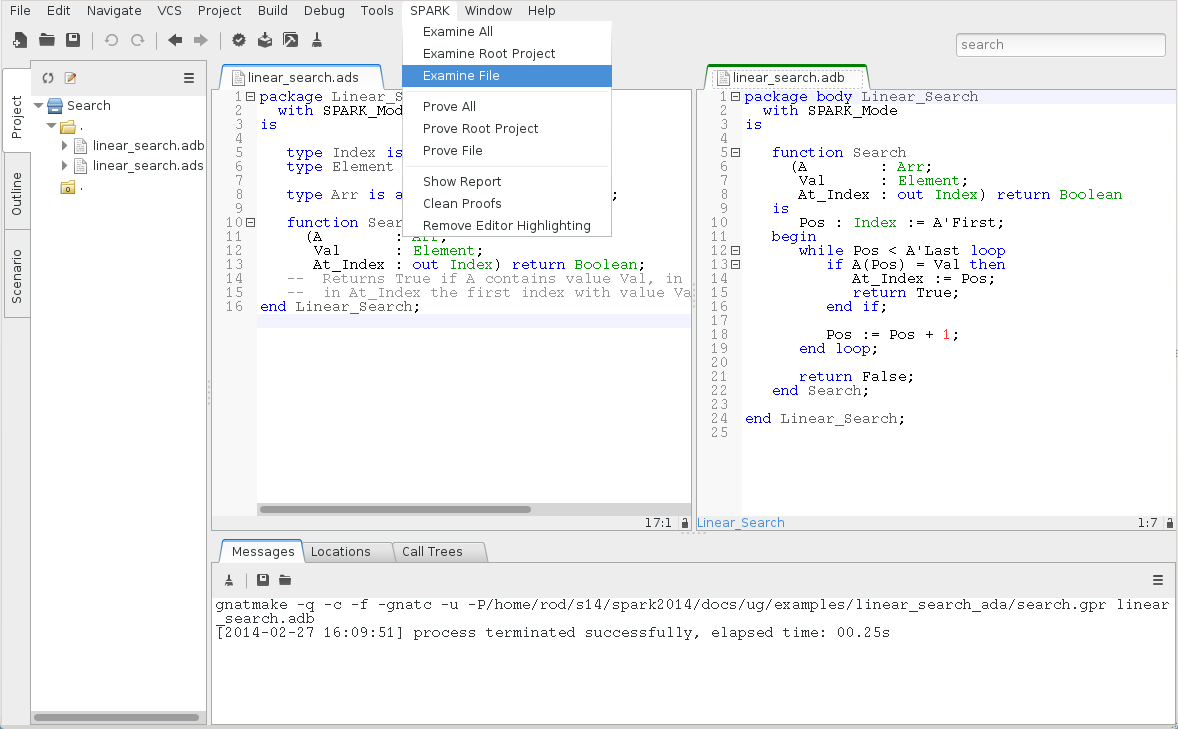It detects here that function `Search` is not in SPARK, because it has an `out` parameter: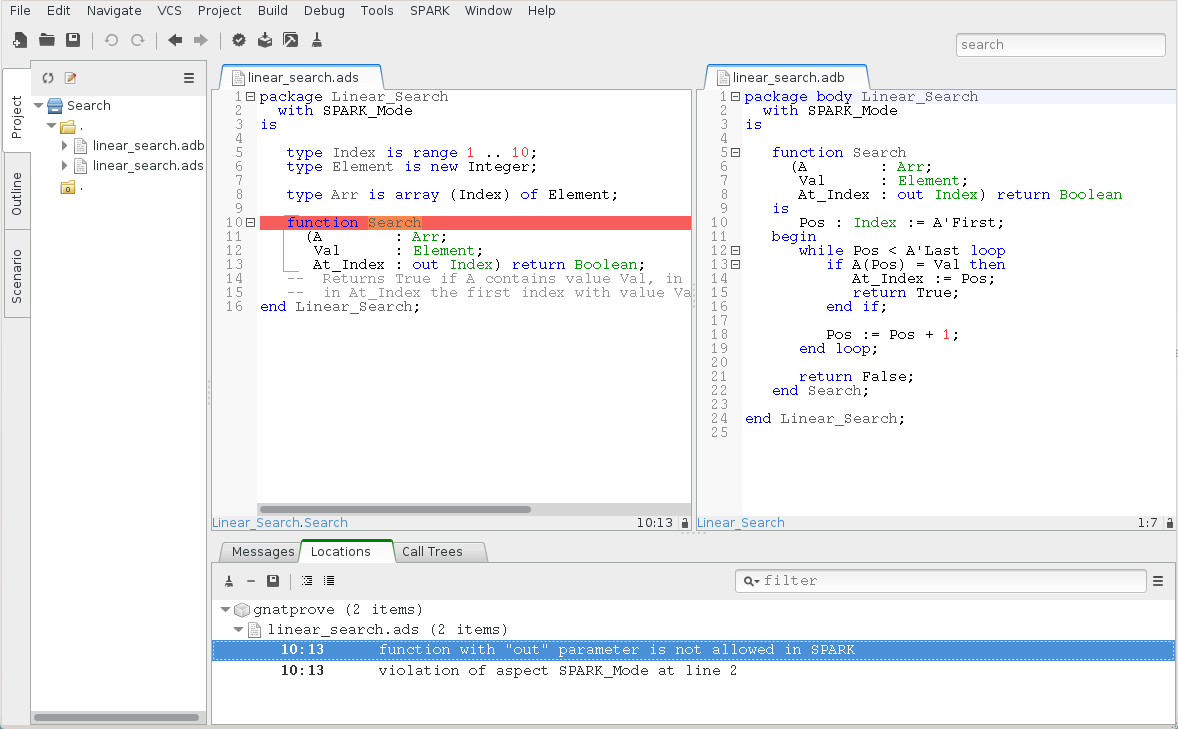The permission in Ada 2012 to have `out` parameters to functions is not allowed in SPARK, because it causes calls to have side-effects (assigning to their `out` parameters), which means that various calls in the same expression may be conflicting, yielding different results depending on the order of evaluation of the expression.

We correct this problem by defining a record type `Search_Result` in `linear_search.ads` holding both the Boolean result and the index for cases when the value is found, and making `Search` return this type:

 ``` 1 2 3 4 5 6 7 8 9 10 11 12 13 14 15 16 17 18 19``` ```package Linear_Search with SPARK_Mode is type Index is range 1 .. 10; type Element is new Integer; type Arr is array (Index) of Element; type Search_Result is record Found : Boolean; At_Index : Index; end record; function Search (A : Arr; Val : Element) return Search_Result; end Linear_Search; ```

The implementation of `Search` in `linear_search.adb` is modified to use this type:

 ``` 1 2 3 4 5 6 7 8 9 10 11 12 13 14 15 16 17 18 19 20 21 22 23 24 25 26``` ```package body Linear_Search with SPARK_Mode is function Search (A : Arr; Val : Element) return Search_Result is Pos : Index := A'First; Res : Search_Result; begin while Pos < A'Last loop if A(Pos) = Val then Res.At_Index := Pos; Res.Found := True; return Res; end if; Pos := Pos + 1; end loop; Res.Found := False; return Res; end Search; end Linear_Search; ```

### 6.1.2. Checking SPARK Initialization Policy¶

Re-running GNATprove on this unit, still using the SPARK ‣ Examine File menu, now reports a different kind of error. This time it is the static analysis pass of GNATprove called flow analysis that detects an attempt of the program to return variable `Res` while it is not fully initialized, thus violating the initialization policy of SPARK: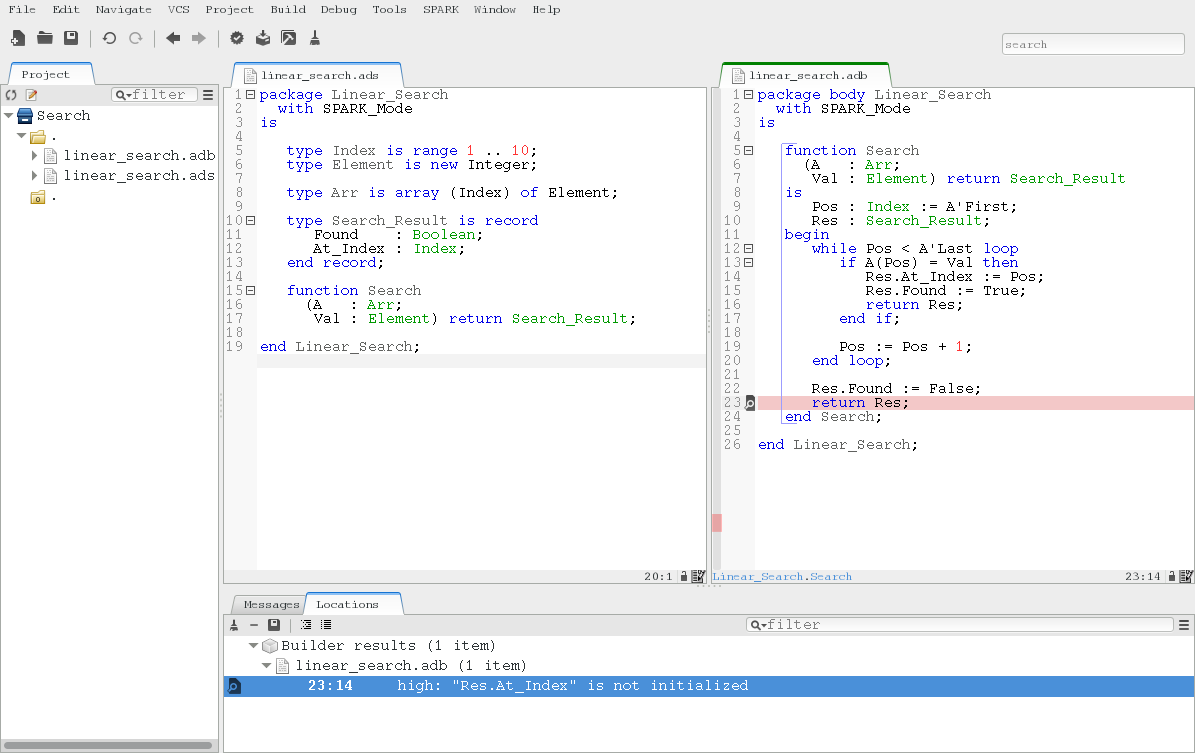Inside the GNAT Studio editor, we can click on the icon, either on the left of the message, or on line 23 in file `linear_search.adb`, to show the path on which `Res.At_Index` is not initialized:Another click on the icon makes the path disappear.

This shows that, when the value is not found, the component `At_Index` of the value returned is indeed not initialized. Although that is allowed in Ada, SPARK requires that all inputs and outputs of subprograms are completely initialized (and the value returned by a function is such an output). As a solution, we could give a dummy value to component `At_Index` when the search fails, but we choose here to turn the type `Search_Result` in `linear_search.ads` into a discriminant record, so that the component `At_Index` is only usable when the search succeeds:

 ```1 2 3 4 5 6 7 8``` ``` type Search_Result (Found : Boolean := False) is record case Found is when True => At_Index : Index; when False => null; end case; end record; ```

Then, in the implementation of `Search` in `linear_search.adb`, we change the value of the discriminant depending on the success of the search:

 ``` 1 2 3 4 5 6 7 8 9 10 11 12 13 14 15 16 17 18 19 20``` ``` function Search (A : Arr; Val : Element) return Search_Result is Pos : Index := A'First; Res : Search_Result; begin while Pos < A'Last loop if A(Pos) = Val then Res := (Found => True, At_Index => Pos); return Res; end if; Pos := Pos + 1; end loop; Res := (Found => False); return Res; end Search; ```

Now re-running GNATprove on this unit, using the SPARK ‣ Examine File menu, shows that there are no reads of uninitialized data.

### 6.1.3. Writing Functional Contracts¶

We now have a valid SPARK program. It is not yet very interesting SPARK code though, as it does not contain any contracts, which are necessary to be able to apply formal verification modularly on each subprogram, independently of the implementation of other subprograms. The precondition constrains the value of input parameters, while the postcondition states desired properties of the result of the function. See Preconditions and Postconditions for more details. Here, we can require in the precondition of `Search` in `linear_search.ads` that callers of `Search` always pass a non-negative value for parameter `Val`, and we can state that, when the search succeeds, the index returned points to the desired value in the array:

 ```1 2 3 4 5 6 7``` ``` function Search (A : Arr; Val : Element) return Search_Result with Pre => Val >= 0, Post => (if Search'Result.Found then A (Search'Result.At_Index) = Val), ```

Notice the use of an if-expression in the postcondition to express an implication: if the search succeeds it implies that the value at the returned index is the value that was being searched for. Note also the use of `Search'Result` to denote the value returned by the function.

This contract is still not very strong. Many faulty implementations of the search would pass this contract, for example one that always fails (thus returning with `Search'Result.Found = False`). We could reinforce the postcondition, but we choose here to do it through a contract by cases, which adds further constraints to the usual contract by precondition and postcondition. We want to consider here three cases:

• the desired value is found at the first index (1)

• the desired value is found at other indexes (2 to 10)

• the desired value is not found in the range 1 to 10

In the first case, we want to state that the index returned is 1. In the second case, we want to state that the search succeeds. In the third case, we want to state that the search fails. We use a helper function `Value_Found_In_Range` in `linear_search.ads` to express that a value `Val` is found in an array `A` within given bounds `Low` and `Up`:

 ``` 1 2 3 4 5 6 7 8 9 10 11 12 13 14 15 16 17 18 19 20``` ``` function Value_Found_In_Range (A : Arr; Val : Element; Low, Up : Index) return Boolean is (for some J in Low .. Up => A(J) = Val); function Search (A : Arr; Val : Element) return Search_Result with Pre => Val >= 0, Post => (if Search'Result.Found then A (Search'Result.At_Index) = Val), Contract_Cases => (A(1) = Val => Search'Result.At_Index = 1, Value_Found_In_Range (A, Val, 2, 10) => Search'Result.Found, (for all J in Arr'Range => A(J) /= Val) => not Search'Result.Found); ```

Note that we express `Value_Found_In_Range` as an expression function, a function whose body consists of a single expression, which can be given in a specification file.

Note also the use of quantified expressions to express properties over collections: `for some` in `Value_Found_In_Range` expresses an existential property (there exists an index in this range such that …), `for all` in the third contract case expresses a universal property (all indexes in this range are such that …).

Each contract case consists of a guard (on the left of the arrow symbol) evaluated on subprogram entry, and a consequence (on the right of the arrow symbol) evaluated on subprogram exit. The special expression `Search'Result` may be used in consequence expressions. The three guards here should cover all possible cases, and be disjoint. When a contract case is activated (meaning its guard holds on entry), its consequence should hold on exit.

The program obtained so far is a valid SPARK program, which GNAT analyzes semantically without errors or warnings.

## 6.2. Testing SPARK Programs¶

We can compile the above program, and test it on a set of selected inputs. The following test program in file `test_search.adb` exercises the case where the searched value is present in the array and the case where it is not:

 ``` 1 2 3 4 5 6 7 8 9 10 11 12 13 14 15 16 17 18 19 20 21 22 23 24 25 26``` ```with Linear_Search; use Linear_Search; with Ada.Text_IO; use Ada.Text_IO; procedure Test_Search is A : constant Arr := (1, 5, 3, 8, 8, 2, 0, 1, 0, 4); Res : Search_Result; begin Res := Search (A, 1); if Res.Found then if Res.At_Index = 1 then Put_Line ("OK: Found existing value at first index"); else Put_Line ("not OK: Found existing value at other index"); end if; else Put_Line ("not OK: Did not find existing value"); end if; Res := Search (A, 6); if not Res.Found then Put_Line ("OK: Did not find non-existing value"); else Put_Line ("not OK: Found non-existing value"); end if; end Test_Search; ```

We can check that the implementation of `Linear_Search` passes this test by compiling and running the test program:

```\$ gnatmake test_search.adb
\$ test_search
> OK: Found existing value at first index
> OK: Did not find non-existing value
```

Note

We use above the command-line interface to compile and run the test program `test_search.adb`. You can do the same inside GNAT Studio by selecting the menu Project ‣ Properties and inside the panel Main of folder Sources, add `test_search.adb` as a main file. Then, click OK. To generate the `test_search` executable, you can now select the menu Build ‣ Project ‣ test_search.adb and to run the `test_search` executable, you can select the menu Build ‣ Run ‣ test_search.

But only part of the program was really tested, as the contract was not checked during execution. To check the contract at run time, we recompile with the switch `-gnata` (`a` for assertions, plus switch `-f` to force recompilation of sources that have not changed):

• a check is inserted that the precondition holds on subprogram entry

• a check is inserted that the postcondition holds on subprogram exit

• a check is inserted that the guards of contract cases are disjoint on subprogram entry (no two cases are activated at the same time)

• a check is inserted that the guards of contract cases are complete on subprogram entry (one case must be activated)

• a check is inserted that the consequence of the activated contract case holds on subprogram exit

Note that the evaluation of the above assertions may also trigger other run-time check failures, like an index out of bounds. With these additional run-time checks, an error is reported when running the test program:

```\$ gnatmake -gnata -f test_search.adb
\$ test_search
> raised SYSTEM.ASSERTIONS.ASSERT_FAILURE : contract cases overlap for subprogram search
```

Note

We use above the command-line interface to add compilation switch `-gnata` and force recompilation with switch `-f`. You can do the same inside GNAT Studio by selecting the menu Project ‣ Properties and inside the panel Ada of the subfolder Switches of folder Build, select the checkbox Enable assertions. Then, click OK. To force recompilation with the new switch, you can now select the menu Build ‣ Clean ‣ Clean All followed by recompilation with Build ‣ Project ‣ test_search.adb. Then run the `test_search` executable with Build ‣ Run ‣ test_search.

It appears that two contract cases for `Search` are activated at the same time! More information can be generated at run time if the code is compiled with the switch `-gnateE`:

```\$ gnatmake -gnata -gnateE -f test_search.adb
\$ test_search
> raised SYSTEM.ASSERTIONS.ASSERT_FAILURE : contract cases overlap for subprogram search
>   case guard at linear_search.ads:33 evaluates to True
>   case guard at linear_search.ads:35 evaluates to True
```

It shows here that the guards of the first and second contract cases hold at the same time. This failure in annotations can be debugged with `gdb` like a failure in the code (provided the program was compiled with appropriate switches, like `-g -O0`). The stack trace inside GNAT Studio shows that the error occurs on the first call to `Search` in the test program: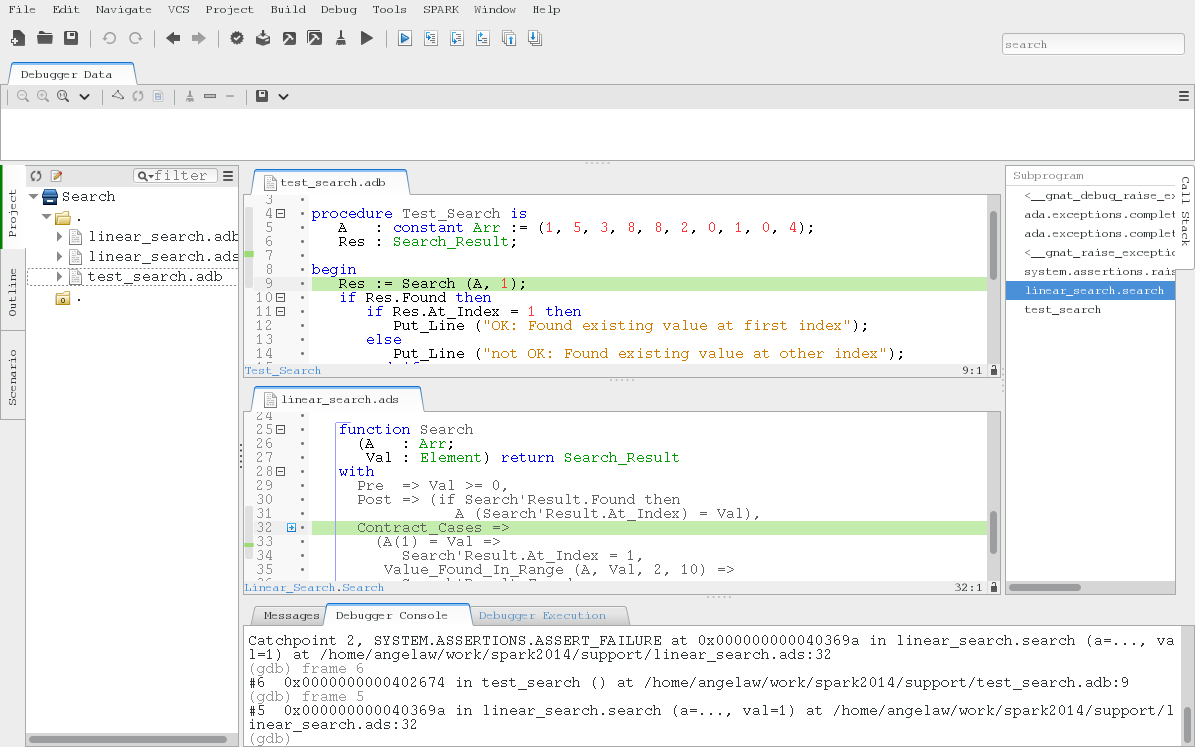Indeed, the value 1 is present twice in the array, at indexes 1 and 8, which makes the two guards `A(1) = Val` and ```Value_Found_In_Range (A, Val, 2, 10)``` evaluate to `True`. We correct the contract of `Search` in `linear_search.ads` by strengthening the guard of the second contract case, so that it only applies when the value is not found at index 1:

 ```1 2 3 4 5 6 7``` ``` Contract_Cases => (A(1) = Val => Search'Result.At_Index = 1, A(1) /= Val and then Value_Found_In_Range (A, Val, 2, 10) => Search'Result.Found, (for all J in Arr'Range => A(J) /= Val) => not Search'Result.Found); ```

With this updated contract, the test passes again, but this time with assertions checked at run time:

```\$ gnatmake -gnata test_search.adb
\$ test_search
> OK: Found existing value at first index
> OK: Did not find non-existing value
```

The program obtained so far passes successfully a test campaign (of one test!) that achieves 100% coverage for all the common coverage criteria, once impossible paths have been ruled out: statement coverage, condition coverage, the MC/DC coverage used in avionics, and even the full static path coverage.

## 6.3. Proving SPARK Programs¶

Formal verification of SPARK programs is a two-step process:

1. the first step checks that flows through the program correctly implement the specified flows (if any), and that all values read are initialized.

2. the second step checks that the program correctly implement its specified contracts (if any), and that no run-time error can be raised.

Step 1 is implemented as a static analysis pass in the tool GNATprove, in `flow` mode. We have seen this flow analysis at work earlier (see Checking SPARK Initialization Policy). Step 2 is implemented as a deductive verification (a.k.a. proof) pass in the tool GNATprove, in the default `all` mode.

The difference between these two steps should be emphasized. Flow analysis in step 1 is a terminating algorithm, which typically takes 2 to 10 times as long as compilation to complete. Proof in step 2 is based on the generation of logical formulas for each check to prove, which are then passed on to automatic provers to decide whether the logical formula holds or not. The generation of logical formulas is a translation phase, which typically takes 10 times as long as compilation to complete. The automatic proof of logical formulas may take a very long time, or never terminate, hence the use of a timeout (1s at proof level 0) for each call to the automatic provers. It is this last step which takes the most time when calling GNATprove on a program, but it is also a step which can be completely parallelized (using switch `-j` to specify the number of parallel processes): each logical formula can be proved independently, so the more cores are available the faster it completes.

Note

The proof results presented in this tutorial may slightly vary from the results you obtain on your machine, as automatic provers may take more or less time to complete a proof depending on the platform and machine used.

Let us continue with our running example. This time we will see how step 2 works to prove contracts and absence of run-time errors, using the main mode `all` of GNATprove reached through the SPARK ‣ Prove File menu.Note

The proof panels presented in this tutorial correspond to an advanced user profile. A simpler proof panel is displayed when the basic user profile is selected (the default). You can switch to the advanced user profile in menu Edit ‣ Preferences ‣ SPARK, by changing the value of User profile from `Basic` to `Advanced`. See Running GNATprove from GNAT Studio for details.

We use the default settings and click on Execute. It completes in a few seconds, with a message stating that some checks could not be proved: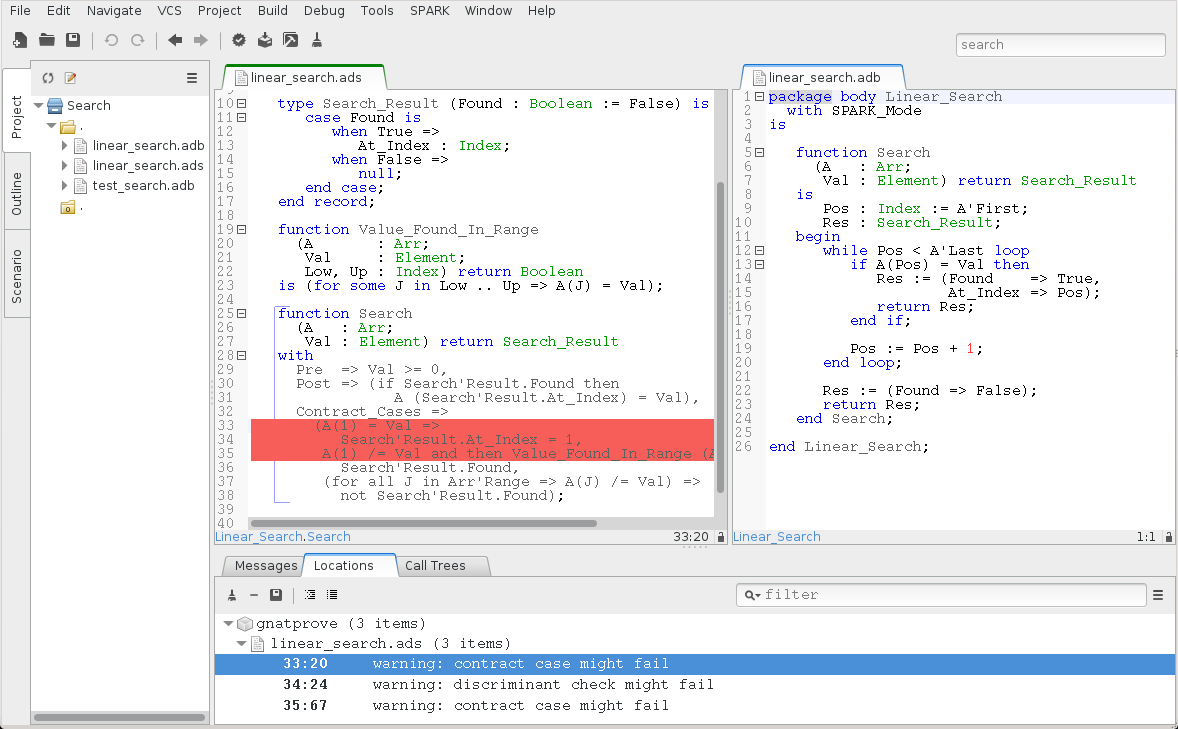Note that there is no such message on the postcondition of `Search`, which means that it was proved. Likewise, there are no such messages on the body of `Search`, which means that no run-time errors can be raised when executing the function.

These messages correspond to checks done when exiting from `Search`. It is expected that not much can be proved at this point, given that the body of `Search` has a loop but no loop invariant, so the formulas generated for these checks assume the worst about locations modified in the loop. A loop invariant is a special pragma `Loop_Invariant` stating an assertion in a loop, which can be both executed at run-time like a regular pragma `Assert`, and used by GNATprove to summarize the effect of successive iterations of the loop. We need to add a loop invariant in `linear_search.adb` stating enough properties about the cumulative effect of loop iterations, so that the contract cases of `Search` become provable. Here, it should state that the value searched was not previously found:

```         pragma Loop_Invariant
(not Value_Found_In_Range (A, Val, A'First, Pos));
```

As stated above, this invariant holds exactly between the two statements in the loop in `linear_search.adb` (after the if-statement, before the increment of the index). Thus, it should be inserted at this place. With this loop invariant, two checks previously not proved are now proved, and a check previously proved becomes unproved: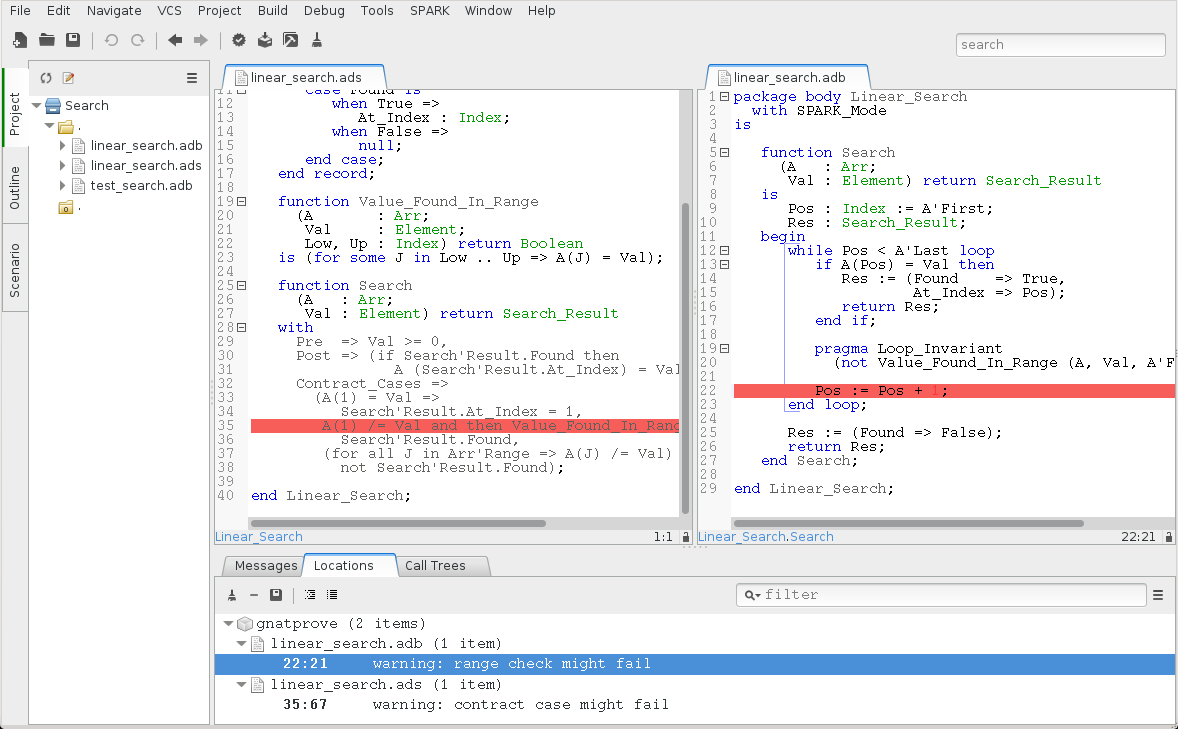The new unproved checks may seem odd, since all we did was add information in the form of a loop invariant. The reason is that we also removed information at the same time. By adding a loop invariant, we require GNATprove to prove iterations around the (virtual) loop formed by the following steps:

1. Take any context satisfying the loop invariant, which summarizes all previous iterations of the loop.

2. Execute the end of a source loop iteration (just the increment here).

3. Test whether the loop exits, and continue with values which do not exit.

4. Execute the start of a source loop iteration (just the if-statement here).

5. Check that the loop invariant still holds.

Around this virtual loop, nothing guarantees that the index `Pos` is below the maximal index at step 2 (the increment), so the range check cannot be proved. It was previously proved because, in the absence of a loop invariant, GNATprove proves iterations around the source loop, and then we get the information that, since the loop did not exit, its test `Pos < A'Last` is false, so the range check can be proved.

We solve this issue by setting the type of `Pos` in `linear_search.adb` to the base type of `Index`, which ranges past the last value of `Index`. (This may not be the simplest solution, but we use it here for the dynamics of this tutorial.)

```      Pos : Index'Base := A'First;
```

And we add the range information for `Pos` to the loop invariant in `linear_search.adb`:

```         pragma Loop_Invariant
(Pos in A'Range
and then
not Value_Found_In_Range (A, Val, A'First, Pos));
```

This allows GNATprove to prove the range check, but not the contract: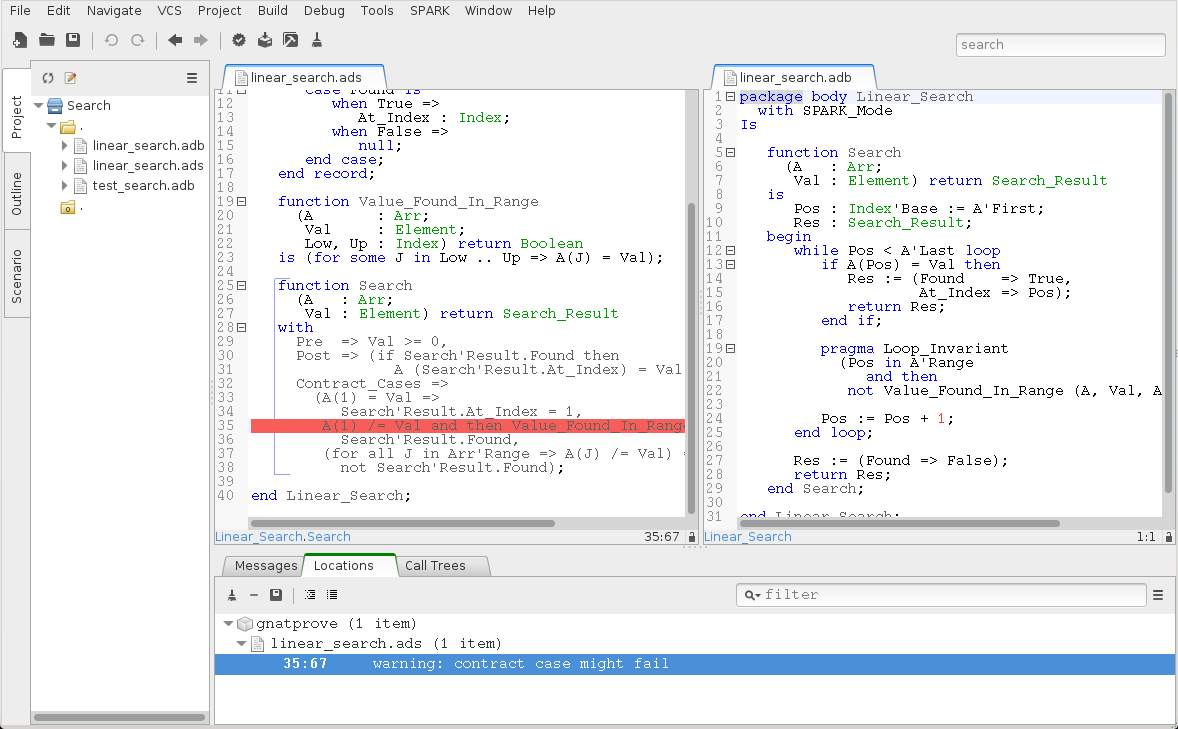This is actually progress! Indeed, the loop invariant should be strong enough to:

1. prove the absence of run-time errors in the loop and after the loop

2. prove that it is preserved from iteration to iteration

3. prove the postcondition and contract cases of the subprogram

So we have just achieved goal 1 above!

As we have modified the code and annotations, it is a good time to compile and run our test program, before doing any more formal verification work. This helps catch bugs early, and it’s easy to do! In particular, the loop invariant will be dynamically checked at each iteration through the loop. Here, testing does not show any problems:

```\$ gnatmake -gnata test_search.adb
\$ test_search
> OK: Found existing value at first index
> OK: Did not find non-existing value
```

The next easy thing to do is to increase the timeout of automatic provers. Its default of 1s is deliberately low, to facilitate interaction with GNATprove during the development of annotations, but it is not sufficient to prove the more complex checks. Let’s increase it to 10s (or equivalently set the ```Proof level``` to 2 in the proof panel corresponding to a basic user profile), and rerun GNATprove: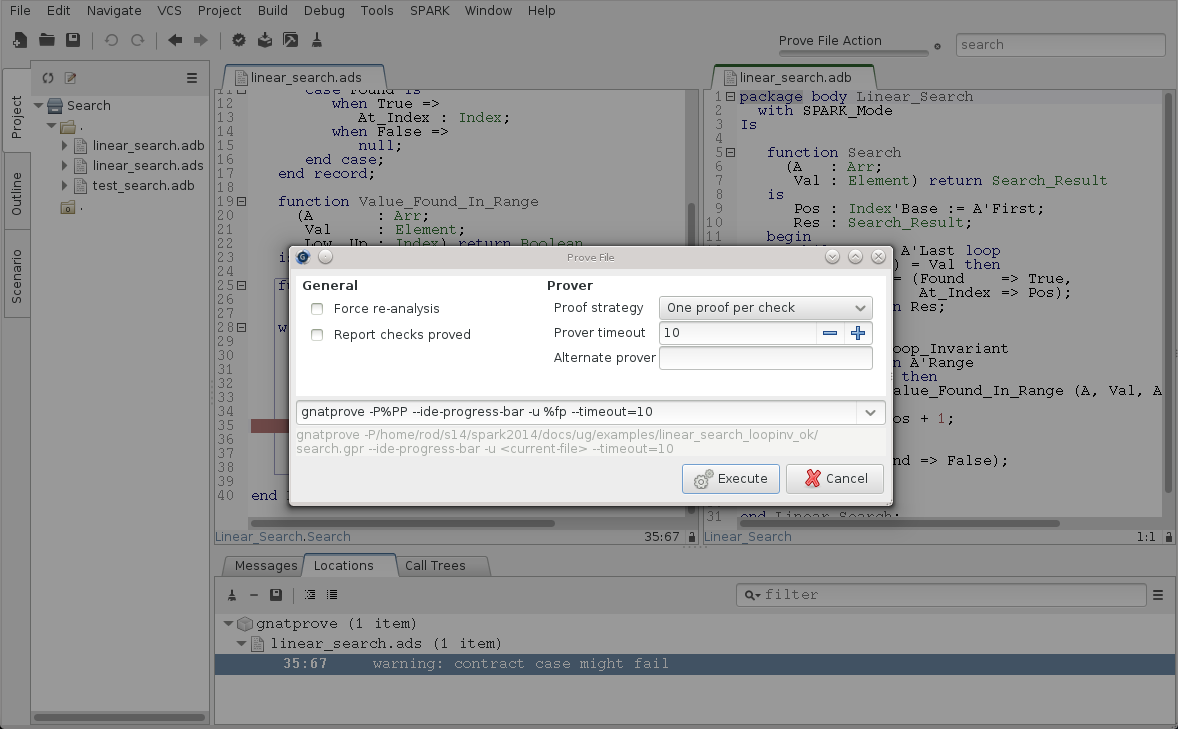The unproved check remains in the contract cases of `Linear_Search`. The next step is to use the SPARK ‣ Prove Line contextual menu available on line 35: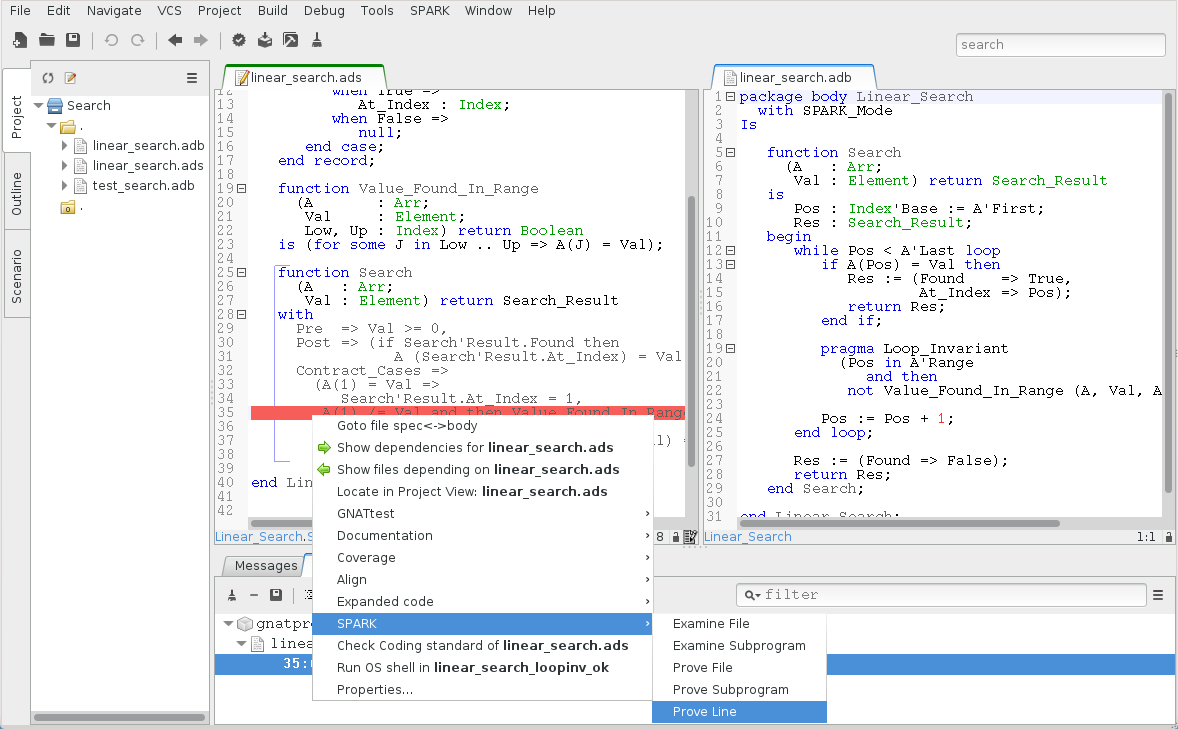We select the `Progressively split` value for choice `Proof strategy` in the window raised in order to maximize proof precision (or equivalently set the `Proof level` to 3 in the proof panel corresponding to a basic user profile), and click on Execute:This runs GNATprove only on the checks that originate from line 35, in a special mode which considers separately individual execution paths if needed. The check is still not proved, but GNAT Studio now displays an icon, either on the left of the message, or on line 35 in file `linear_search.ads`, to show the path on which the contract case is not proved:This corresponds to a case where the implementation of `Search` does not find the searched value, but the guard of the second contract case holds, meaning that the value is present in the range 2 to 10. Looking more closely at the path highlighted, we can see that the loop exits when `Pos = A'Last`, so the value 10 is never considered! We correct this bug by changing the loop test in `linear_search.adb` from a strict to a non-strict comparison operation:

```      while Pos <= A'Last loop
```

On this modified code, we rerun GNATprove on line 35, checking the box `Report checks proved` to get information even when a check is proved. The reassuring green color (and the accompanying info message) show that the check was proved this time:As usual after code changes, we rerun the test program, which shows no errors. Rerunning GNATprove on the complete file shows no more unproved checks. The `Linear_Search` unit has been fully proved. To see all the checks that were proved, we can rerun the tool with box `Report checks proved` checked, which displays the results previously computed: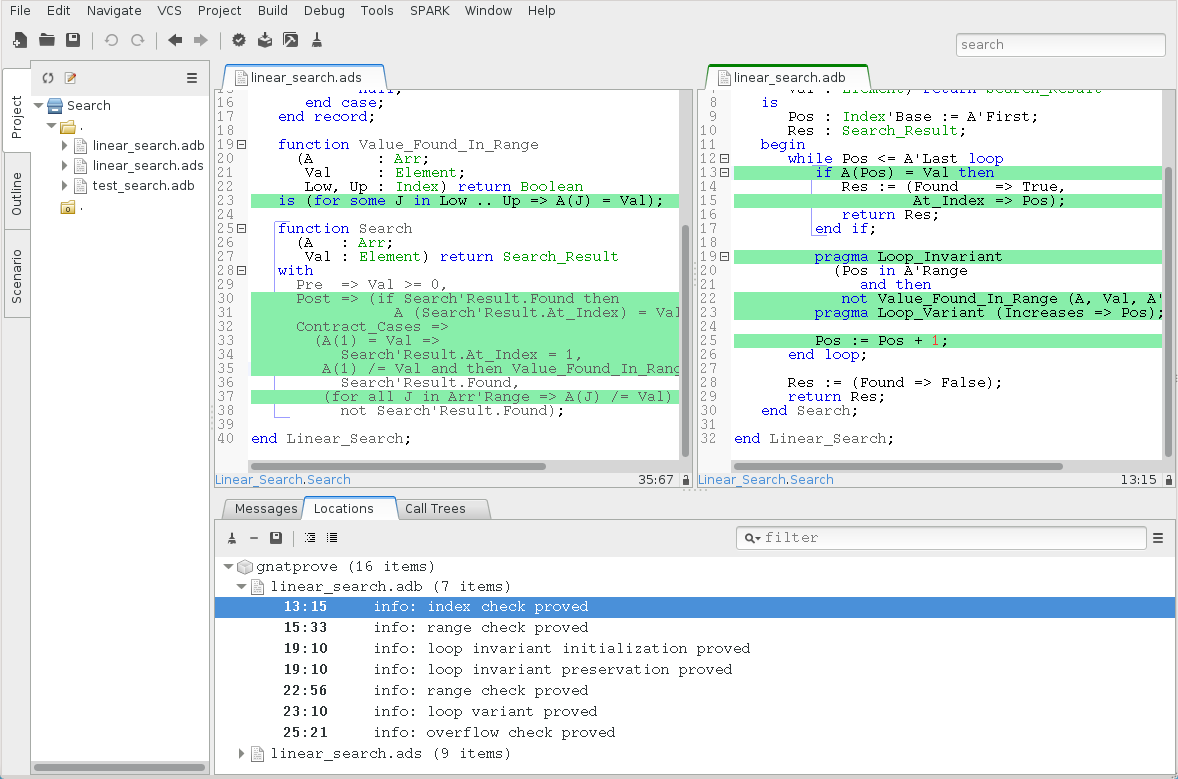Note that one thing that was not proved is that `Search` terminates. As it contains a while-loop, it could loop forever. To prove that it is not the case, we add a loop variant, which specifies a quantity varying monotonically with each iteration. Since this quantity is bounded by its type, and we have proved absence of run-time errors in `Search`, proving this monotonicity property also shows that there cannot be an infinite number of iterations of the loop. The natural loop variant for `Search` is the index `Pos`, which increases at each loop iteration:

```         pragma Loop_Variant (Increases => Pos);
```

With this line inserted after the loop invariant in `linear_search.adb`, the test program still runs without errors (it checks dynamically that the loop variant is respected), and the program is still fully proved. Here is the final version of `Linear_Search`, with the complete annotations:

 ``` 1 2 3 4 5 6 7 8 9 10 11 12 13 14 15 16 17 18 19 20 21 22 23 24 25 26 27 28 29 30 31 32 33 34 35 36 37 38 39 40``` ```package Linear_Search with SPARK_Mode is type Index is range 1 .. 10; type Element is new Integer; type Arr is array (Index) of Element; type Search_Result (Found : Boolean := False) is record case Found is when True => At_Index : Index; when False => null; end case; end record; function Value_Found_In_Range (A : Arr; Val : Element; Low, Up : Index) return Boolean is (for some J in Low .. Up => A(J) = Val); function Search (A : Arr; Val : Element) return Search_Result with Pre => Val >= 0, Post => (if Search'Result.Found then A (Search'Result.At_Index) = Val), Contract_Cases => (A(1) = Val => Search'Result.At_Index = 1, A(1) /= Val and then Value_Found_In_Range (A, Val, 2, 10) => Search'Result.Found, (for all J in Arr'Range => A(J) /= Val) => not Search'Result.Found); end Linear_Search; ```
 ``` 1 2 3 4 5 6 7 8 9 10 11 12 13 14 15 16 17 18 19 20 21 22 23 24 25 26 27 28 29 30 31 32``` ```package body Linear_Search with SPARK_Mode is function Search (A : Arr; Val : Element) return Search_Result is Pos : Index'Base := A'First; Res : Search_Result; begin while Pos <= A'Last loop if A(Pos) = Val then Res := (Found => True, At_Index => Pos); return Res; end if; pragma Loop_Invariant (Pos in A'Range and then not Value_Found_In_Range (A, Val, A'First, Pos)); pragma Loop_Variant (Increases => Pos); Pos := Pos + 1; end loop; Res := (Found => False); return Res; end Search; end Linear_Search; ```

The final version of the `linear_search` example is part of the Examples in the Toolset Distribution. This concludes our tutorial on the SPARK toolset.